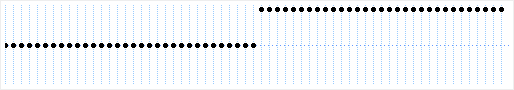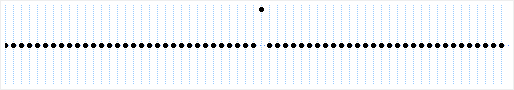# 8. First Difference

Home | Next

A solution to the problem with adding steps is to store the differences between samples into the sound buffer, rather than the absolute values of samples. First, consider a sampled step (not band-limited):Sampled step

The problem is that the right-hand side never ends. This can be eliminated by storing the differences between samples, called the first difference. In this representation a sample is only non-zero when the waveform is changing at that point; since the right-hand side of a step never changes, the ends of its first difference can be cut off without losing anything important (that is, non-zero values):First difference of sampled step

A running sum converts the first difference back to absolute sample points. Each output point is the sum of all previous input points. In the above example the samples around the middle of the first difference are 0, 0, 1, 0, 0. A running sum can be applied as shown below:

```    sum = sum + 0; sum = 0
sum = sum + 0; sum = 0
sum = sum + 1; sum = 1
sum = sum + 0; sum = 1
sum = sum + 0; sum = 1
```